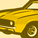# New to Qlik Sense

If you’re new to Qlik Sense, start with this Discussion Board and get up-to-speed quickly.

Announcements
Qlik Cloud Maintenance is scheduled between March 27-30. Visit Qlik Cloud Status page for more details.
cancel
Showing results for
Did you mean:Contributor III

## Count set Analysis measures Aggr function

Hi All,

I want a measure where i need to find Count of  field1 on basis of basis another filed2  value which is greater than some value ?

**Field1 is dimension1  && Field2 is Amount field

I tried below measure but it is wrong

Count(  {<[FISCAL YEAR]=,[FISCAL MONTH]=,[FISCAL QUARTER]=,
DATE={">=\$(=Date(monthstart(today())))<=\$(=Date(today()))"}

, field2 >{'100'}>}  distinct field1)

Labels (5)

• ### Set Analysis

1 Solution

Accepted SolutionsSpecialist II

Perhaps like this:

Count( {<[FISCAL YEAR]=,[FISCAL MONTH]=,[FISCAL QUARTER]=,
DATE={">=\$(=Date(monthstart(today())))<=\$(=Date(today()))"}

,field2 ={'>100'} >} distinct field1)Specialist II

Perhaps like this:

Count( {<[FISCAL YEAR]=,[FISCAL MONTH]=,[FISCAL QUARTER]=,
DATE={">=\$(=Date(monthstart(today())))<=\$(=Date(today()))"}

,field2 ={'>100'} >} distinct field1)Tags
Community Browser